## The Substitution Rule

In first quarter calculus you learned numerous methods for computing derivatives of functions. For example, the power rule asserts that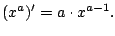We can turn this into a way to compute certain integrals: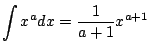if $a&ne#neq;-1$Just as with the power rule, many other rules and results that you already know yield techniques for integration. In general integration is potentially much trickier than differentiation, because it is often not obvious which technique to use, or even how to use it. Integration is a more exciting than differentiation!

Recall the chain rule, which asserts that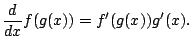We turn this into a technique for integration as follows:

Proposition 2.3.1 (Substitution Rule)   Let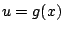, we have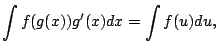assuming that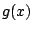is a function that is differentiable and whose range is an interval on which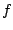is continuous.

Proof. Sinceis continuous on the range of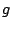, Theorem 2.1.5 (the fundamental theorem of Calculus) implies that there is a function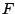such that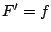. Then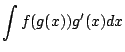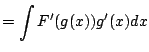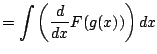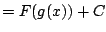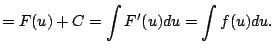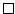Ifthen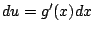, and the substitution rule simply says if you letformally in the integral everywhere, what you naturally would hope to be true based on the notation actually is true. The substitution rule illustrates how the notation Leibniz invented for Calculus is incredibly brilliant. It is said that Leibniz would often spend days just trying to find the right notation for a concept. He succeeded.

As with all of Calculus, the best way to start to get your head around a new concept is to see severally clearly worked out examples. (And the best way to actually be able to use the new idea is to do lots of problems yourself!) In this section we present examples that illustrate how to apply the substituion rule to compute indefinite integrals.

Example 2.3.2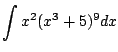Let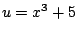. Then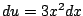, hence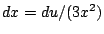. Now substitute it all in: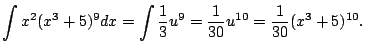There's no point in expanding this out: only simplify for a purpose!''

Example 2.3.3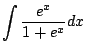Substitute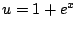. Then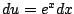, and the integral above becomes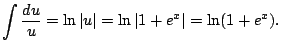Note that the absolute values are not needed, since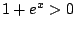for all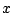.

Example 2.3.4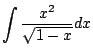Keeping in mind the power rule, we make the substitution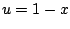. Then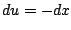. Noting that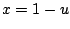by solving forin, we see that the above integral becomes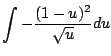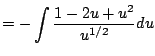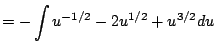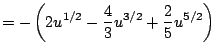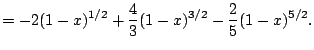William Stein 2006-03-15